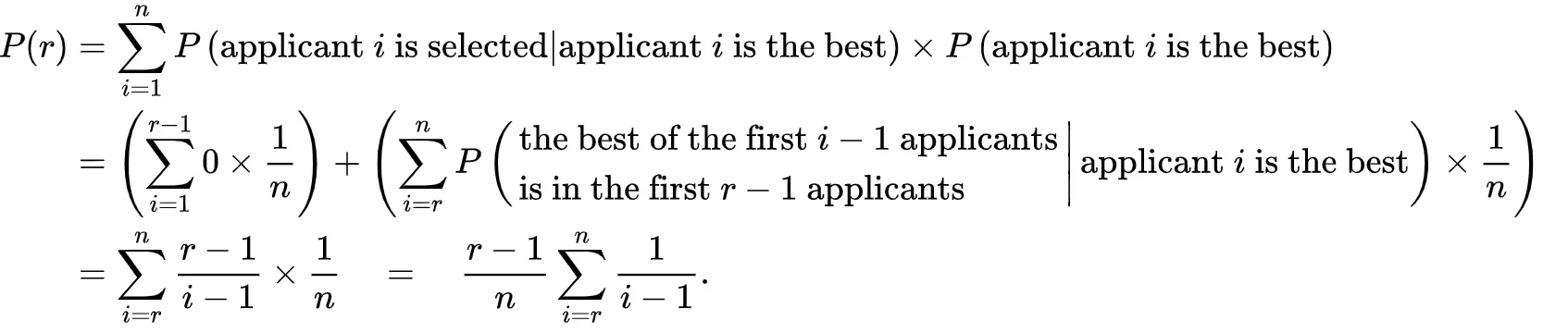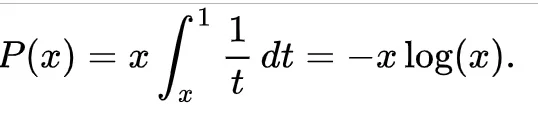# 过年走亲戚应答指南（数学版）——最优截至策略

## How to answer the questions from elders

Posted by qingshan on January 18, 2019

### 提出问题：数学家的秘书问题

1949年，一个名叫Merrill M. Flood的美国数学家，遇到了和当时那个年轻人同样的问题，只不过，这次他的问题更加的具象化了一点，简直就是为了现在年轻人量身定做的一般。问题描述如下：

### 解决：数学建模

• 当设定前 5 个作为样本, 选中最优值的概率是15.625 %
• 当设定前 10 个作为样本, 选中最优值的概率是23.401 %
• 当设定前 15 个作为样本, 选中最优值的概率是28.82 %
• 当设定前 20 个作为样本, 选中最优值的概率是32.682 %
• 当设定前 25 个作为样本, 选中最优值的概率是35.069 %
• 当设定前 30 个作为样本, 选中最优值的概率是36.452 %
• 当设定前 35 个作为样本, 选中最优值的概率是36.744 %
• 当设定前 40 个作为样本, 选中最优值的概率是36.67 %
• 当设定前 45 个作为样本, 选中最优值的概率是36.09 %
• 当设定前 50 个作为样本, 选中最优值的概率是34.957 %
• 当设定前 55 个作为样本, 选中最优值的概率是33.125 %
• 当设定前 60 个作为样本, 选中最优值的概率是30.937 %
• 当设定前 65 个作为样本, 选中最优值的概率是28.168 %
• 当设定前 70 个作为样本, 选中最优值的概率是25.161 %
• 当设定前 75 个作为样本, 选中最优值的概率是21.472 %
• 当设定前 80 个作为样本, 选中最优值的概率是18.099 %
• 当设定前 85 个作为样本, 选中最优值的概率是13.721 %
• 当设定前 90 个作为样本, 选中最优值的概率是9.476 %
• 当设定前 95 个作为样本, 选中最优值的概率是4.8 %
• 当设定前 100 个作为样本, 选中最优值的概率是1.051 %

（为了控制计算时间，在程序中将N设为了100）

**（限于篇幅，文末可以获取完整程序代码）**

### 证明：数学论证### 究极结论### 题外拓展：什么是自然常数e？

• 鹦鹉螺的贝壳像等角螺线
• 菊的种子排列成等角螺线
• 鹰以等角螺线的方式接近它们的猎物
• 飞蛾等昆虫以等角螺线的方式接近光源
• 蜘蛛网的构造与等角螺线相似
• 旋涡星系的旋臂差不多是等角螺线。银河系的四大旋臂的倾斜度约为 12°
• 低气压(热带气旋、温带气旋等)的外观像等角螺线### 附录：程序代码

1
2
3
from __future__ import division
import numpy as np
import matplotlib.pyplot as plt

1
2
3
4
5
6
7
8
9
10
11
12
13
14
15
16
17
18
19
20
21
22
def choose_candidate(n, reject=np.e):
'''Choose a candidate from a list of n candidates using
a specified strategy.

reject: percentage of candidates to initially reject (optimal strategy by default)
'''

candidates = np.arange(1, n+1)
np.random.shuffle(candidates)

if reject == np.e:
stop = int(round(n/reject))
else:
stop = int(round(reject*n/100))

best_from_rejected = np.min(candidates[:stop])
rest = candidates[stop:]

try:
return rest[rest < best_from_rejected]
except IndexError:
return candidates[-1]

1
2
3
4
5
6
7
8
9
10
# choose from 100 candidates and run simulation 100,000 times
sim = np.array([choose_candidate(n=100) for i in range(100000)])

plt.figure(figsize=(10, 6))
plt.hist(sim, bins=100)
plt.xticks(np.arange(0, 101, 10))
plt.ylim(0, 40000)
plt.xlabel('Chosen candidate')
plt.ylabel('frequency')
plt.show()

1
2
3
4
5
6
7
8
9
plt.figure(figsize=(10, 6))
plt.plot(np.cumsum(np.histogram(sim, bins=100))/100000)
plt.ylim(0,1)
plt.xlim(0, 100)
plt.yticks(np.arange(0, 1.1, 0.1))
plt.xticks(np.arange(0, 101, 10))
plt.xlabel('Chosen candidate')
plt.ylabel('Cumulative probability')
plt.show()

1
2
3
4
5
6
7
8
9
10
11
12
13
14
15
16
best_candidate = []
for r in range(5, 101, 5):
sim = np.array([choose_candidate(n=100, reject=r) for i in range(100000)])
# np.histogram counts frequency of each candidate
best_candidate.append(np.histogram(sim, bins=100)/100000)

plt.figure(figsize=(10, 6))
plt.scatter(range(5, 101, 1), best_candidate)
plt.xlim(0, 100)
plt.xticks(np.arange(0, 101, 10))
plt.ylim(0, 0.4)
plt.xlabel('% of candidates rejected')
plt.ylabel('Probability of choosing best candidate')
plt.grid(True)
plt.axvline(100/np.e, ls='--', c='black')
plt.show()

1
2
for i in xrange(len(best_candidate)):
print(u"当设定前 {} 个作为样本, 选中最优值的概率是{} %".format((i+1)*5, best_candidate[i] * 100))

1
2
3
4
5
6
7
8
9
10
def get_best_candidates(best_n=1):
'''Return a list of probabilities for different rejection strategies and specify what percentage of the
best candidates we want to select.'''

best_candidate = []
for c in  + range(5, 101, 5):
sim = np.array([choose_candidate(100, reject=c) for i in range(10000)])
best_candidate.append(len(sim[sim <= best_n])/100)

return best_candidate

1
2
3
4
5
6
7
8
9
10
11
12
13
plt.figure(figsize=(10, 6))
for i in [1, 2, 5, 10]:
plt.scatter(range(0, 101, 5), get_best_candidates(i), label=str(i))
plt.xlim(0, 100)
plt.ylim(0, 100)
plt.xticks(np.arange(0, 101, 5))
plt.yticks(np.arange(0, 101, 10))
plt.xlabel('% of candidates rejected')
plt.ylabel('Probability of choosing best candidates')
plt.legend(title='No. of best candidates')
plt.grid(True)
plt.tight_layout()
plt.show()